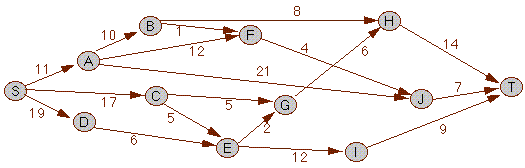## Example: The Stagecoach Problem

This is an example of efficient prototyping of recurrences applied to a dynamic programming problem.

The stagecoach problem is a classical problem in dynamic programming. The background for this problem is said to be a salesman in the wild west. He was travelling from a small town S to another small town T, but did not want to pay more for the transport than necessary. The means of transportation was stagecoaches, that were scheduled between the small towns in the area. The cost of travel was to be found by adding together the cost of the individual distances. Given a schematic map of the possible routes in the form of the graph below with the costs of the individual stretches associated with the arcs, the problem can be formulated as finding the total cost of the cheapest path from S to T in the graph.The task of finding the cost of cheapest path may be formulated as a recurrence. First we define the graph with the function `cost(p,n)` as giving the cost associated with the arc from town p to town n. Now the function `cheapest(p)` is to give the total, cheapest cost of going from p to the end node T. This is found by looking at all arcs from the node p to a node n, and then adding the `cost(p,n)` to the cheapest cost of going from n to T. The lowest cost from p is then the minimum of all the costs found.

cheapest(p) = min{cost(p,n) + cheapest(n) for all n there is an arc from p to n}

cheapest(T) = 0

In dynamic programming this recurrence will be analysed in order to create a program that will compute the cheapest path in polynomial time, rather than the exponential time given by normal recursive execution of the code. Using our technique, the recurrence will give a polynomial time program directly, eliminating the need to manually develop a non-recursive version.

This was an example of efficient prototyping of recurrences.
© 1997 Magne Haveraaen, University of Bergen, Norway.